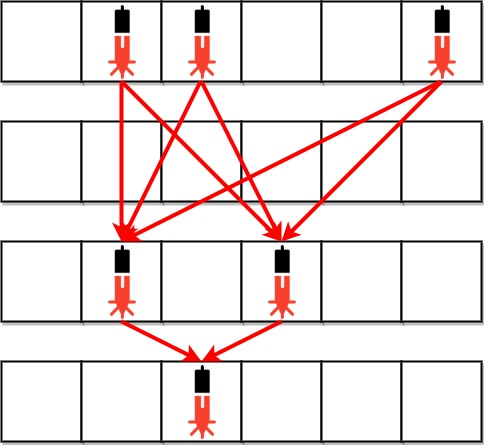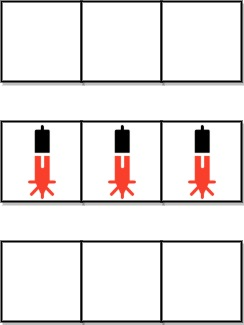Formatted question description: https://leetcode.ca/all/2125.html

# 2125. Number of Laser Beams in a Bank (Medium)

Anti-theft security devices are activated inside a bank. You are given a 0-indexed binary string array bank representing the floor plan of the bank, which is an m x n 2D matrix. bank[i] represents the ith row, consisting of '0's and '1's. '0' means the cell is empty, while'1' means the cell has a security device.

There is one laser beam between any two security devices if both conditions are met:

• The two devices are located on two different rows: r1 and r2, where r1 < r2.
• For each row i where r1 < i < r2, there are no security devices in the ith row.

Laser beams are independent, i.e., one beam does not interfere nor join with another.

Return the total number of laser beams in the bank.

Example 1:Input: bank = ["011001","000000","010100","001000"]
Output: 8
Explanation: Between each of the following device pairs, there is one beam. In total, there are 8 beams:
* bank -- bank
* bank -- bank
* bank -- bank
* bank -- bank
* bank -- bank
* bank -- bank
* bank -- bank
* bank -- bank
Note that there is no beam between any device on the 0th row with any on the 3rd row.
This is because the 2nd row contains security devices, which breaks the second condition.


Example 2:Input: bank = ["000","111","000"]
Output: 0
Explanation: There does not exist two devices located on two different rows.


Constraints:

• m == bank.length
• n == bank[i].length
• 1 <= m, n <= 500
• bank[i][j] is either '0' or '1'.

Similar Questions:

## Solution 1.

// OJ: https://leetcode.com/problems/number-of-laser-beams-in-a-bank/
// Time: O(MN)
// Space: O(1)
class Solution {
public:
int numberOfBeams(vector<string>& A) {
int N = A.size(), prev = -1, ans = 0;
for (auto &s : A) {
int cnt = 0;
for (int i = 0; i < N; ++i) {
cnt += s[i] == '1';
}
if (cnt == 0) continue;
if (prev != -1) ans += prev * cnt;
prev = cnt;
}
return ans;
}
};